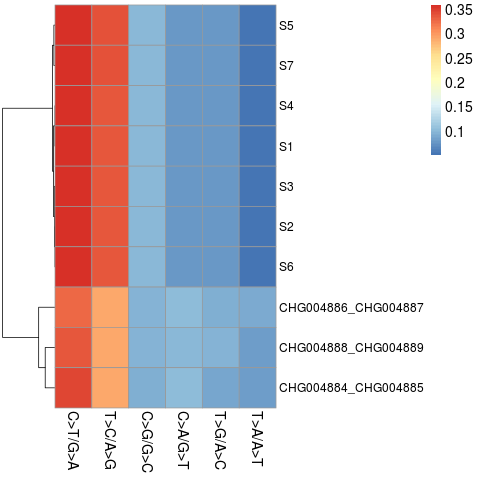# 1，突变频谱数据准备

```Name    Type
S5      T>C/A>G
S5      T>C/A>G
S5      C>T/G>A
S5      T>A/A>T
S5      T>C/A>G
S1      C>T/G>A
S1      T>C/A>G
S1      C>T/G>A
S1      T>C/A>G```

# 2，生成突变频谱图

```library(ggplot2)
f1<- ggplot(data = data1, aes(x = Name, fill = Type)) + geom_bar(position = "fill") + labs(title = "Mutation Spectrum",x = "",y = "Fraction of Mutations") + theme(panel.background = element_blank(), axis.text.x  = element_text(angle=90), text = element_text(size=16) )
svg(file="mutation_spectrum.svg")
f1
dev.off()```# 3，热图数据准备

```Name    C>T/G>A T>C/A>G C>G/G>C C>A/G>T T>G/A>C T>A/A>T
S5      0.3574  0.3383  0.0977  0.0771  0.0776  0.0519
CHG004886_CHG004887     0.3271  0.2890  0.0960  0.1025  0.0942  0.0913```

# 4，绘制热图和聚类

```library(pheatmap)
row.names(data2) <- data2\$Name
data2=data2[,2:7]
data2_matrix<- data.matrix(data2)
png(file="heatmap.png")
pheatmap(data2_matrix,fontsize=14,fontsize_row=12,cluster_rows=T,cluster_cols=F)
dev.off()```#####################################################################
#版权所有 转载请告知 版权归作者所有 如有侵权 一经发现 必将追究其法律责任
#Author: Jason
####################################################################

## 《样本突变频谱分析和基于突变频谱的热图聚类》有2个想法

1.Sarah Zhao

作者很用心。点赞

回复
2.zengda

不错，不错，看看了！

回复